# 20. Draw the orbital notation beyond the noble gas core for osmium. 20. Draw the orbital notation beyond the noble gas core for osmium.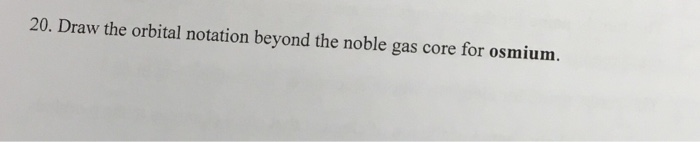20. Draw the orbital notation beyond the noble gas core for osmium.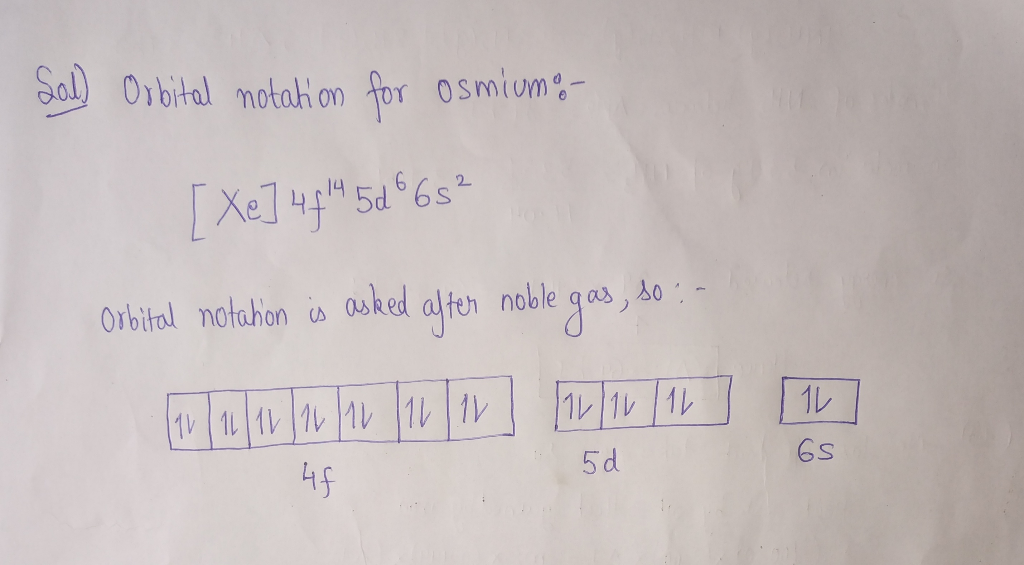##### Add Answer of: 20. Draw the orbital notation beyond the noble gas core for osmium. 20. Draw the orbital notation beyond the noble gas core for osmium.
Similar Homework Help Questions
• ### Write the electron configuration for Se using the noble gas core format

Write the electron configuration for Se using the noble gas core format.

• ### Write the ground state electron configuration of Ca using the noble-gas shorthand notation.

Write the ground state electron configuration of Ca using the noble-gas shorthand notation.

• ### 6. Give the electron configuration (use inert gas symbol for core electrons) and orbital diagram (for valence electrons)...

6. Give the electron configuration (use inert gas symbol for core electrons) and orbital diagram (for valence electrons) and predict the magnetism for each of the following: Br− Cr3+ Ag+ Au+ Cu Fe2+

• ### Using SPECTROSCOPIC notation write the complete electron configuration for the chromium(II) ion. Using NOBLE GAS...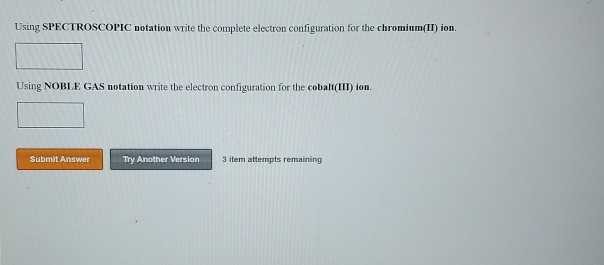Using SPECTROSCOPIC notation write the complete electron configuration for the chromium(II) ion. Using NOBLE GAS notation write the electron configuration for the cobalt(III) ion. Submit Answer Try Another Version 3 item attempts remaining Write ground-state electron configurations for the ions S, P3, Na and Crt. Which do you expect will be paramagnetic due to the presence of unpaired electrons? (Express your answer as a series of orbitals. For example, the electron configuration of Li would be entered in complete form...

• ### Draw the molecular orbital (MO) electron diagram for the Li2 molecule. Be sure your diagram contains...

Draw the molecular orbital (MO) electron diagram for the Li2 molecule. Be sure your diagram contains all of the electrons in the molecule, including any core electrons. Energy

• ### Problem 15. (6 points) Xenon trioxide is a slightly stable molecule that contains a noble gas. Write the electron do...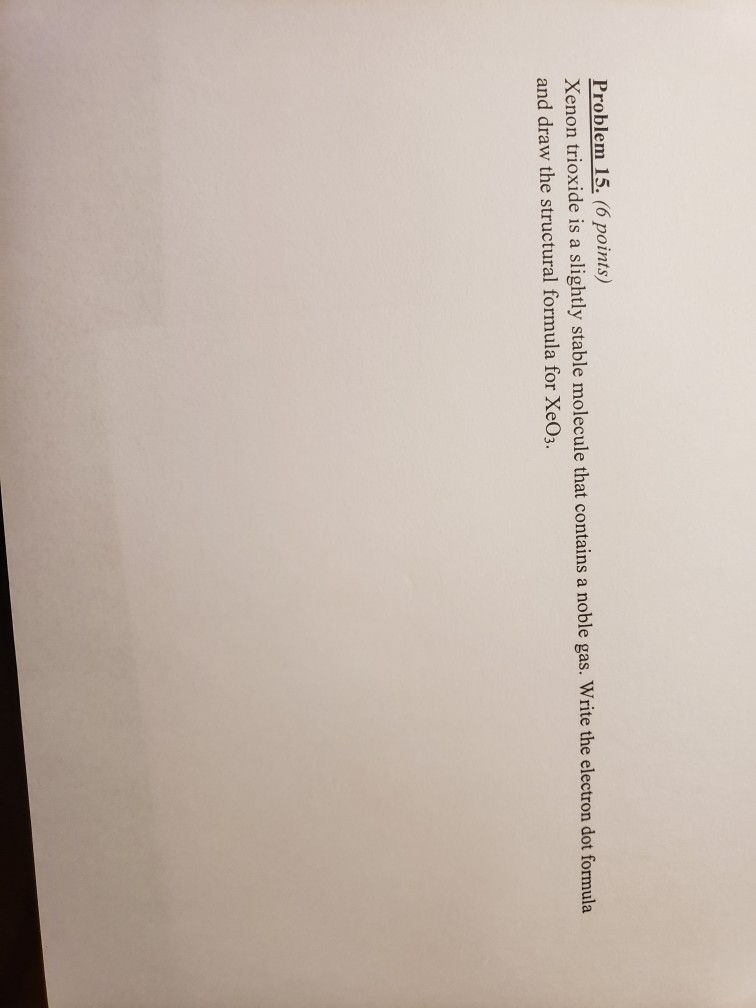Problem 15. (6 points) Xenon trioxide is a slightly stable molecule that contains a noble gas. Write the electron dot formula and draw the structural formula for Xe03.

• ### can someone please answer this in this format of just squares thanks Draw the molecular orbital...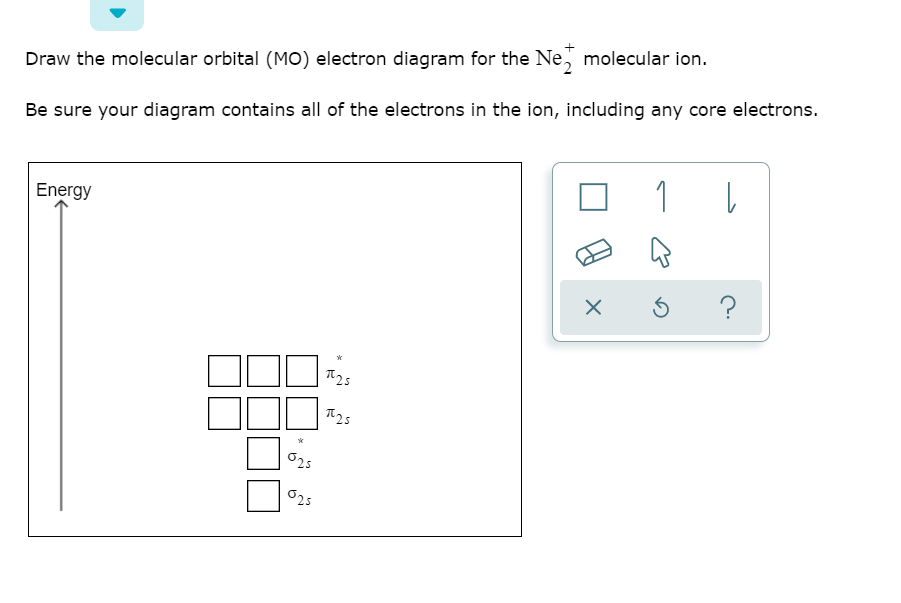can someone please answer this in this format of just squares thanks Draw the molecular orbital (MO) electron diagram for the Ne, molecular ion. Be sure your diagram contains all of the electrons in the ion, including any core electrons. Energy 0 1 [ x 6 ? D002 00012

• ### stands for a noble-gas core and n, m, or o stand for integer numbers like 1, 2, 3 Some patterns of electron configurati...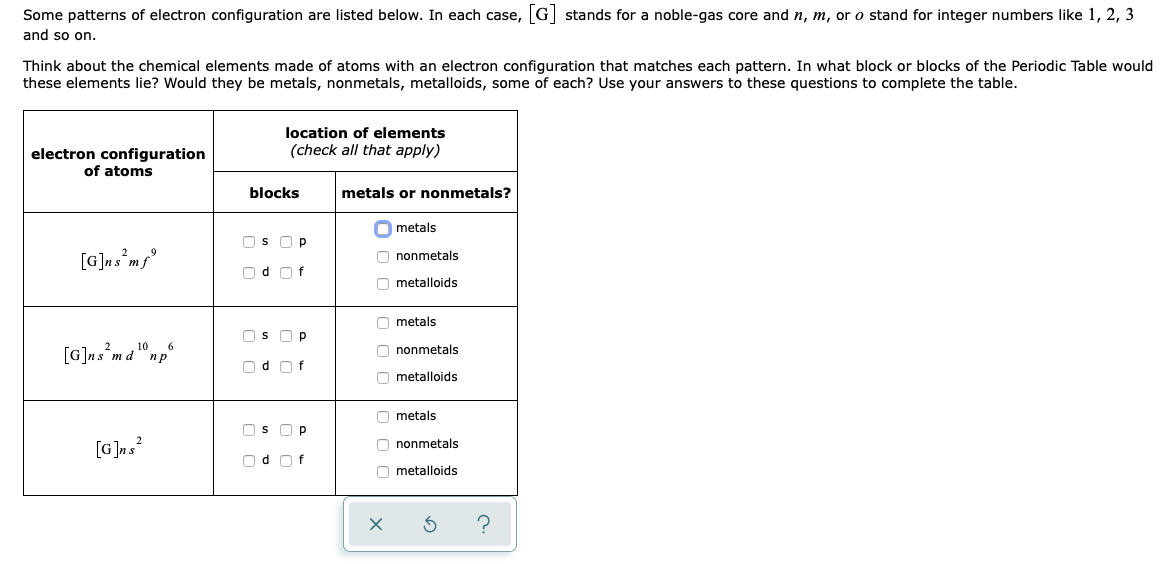stands for a noble-gas core and n, m, or o stand for integer numbers like 1, 2, 3 Some patterns of electron configuration are listed below. In each case, G and so on. Think about the chemical elements made of atoms with an electron configuration that matches each pattern. In what block or blocks of the Periodic Table would these elements lie? Would they be metals, nonmetals, metalloids, some of each? Use your answers to these questions to complete the...

• ### 20. Draw a Lewis Dot Structure of 1,2-dibromo-ethylene (C,BraH) (one bromine per carbon). Use Hybrid Orbital theory to show the type of hybridized orbital sets and non-hybridized orbitals for eac...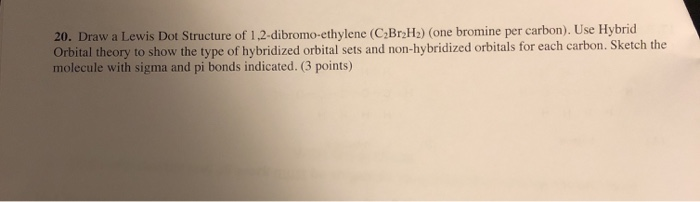20. Draw a Lewis Dot Structure of 1,2-dibromo-ethylene (C,BraH) (one bromine per carbon). Use Hybrid Orbital theory to show the type of hybridized orbital sets and non-hybridized orbitals for each carbon. Sketch the molecule with sigma and pi bonds indicated. (3 points) 20. Draw a Lewis Dot Structure of 1,2-dibromo-ethylene (C,BraH) (one bromine per carbon). Use Hybrid Orbital theory to show the type of hybridized orbital sets and non-hybridized orbitals for each carbon. Sketch the molecule with sigma and pi...

• ### The last question is what is the atomic number of the second noble gas? Suppose you...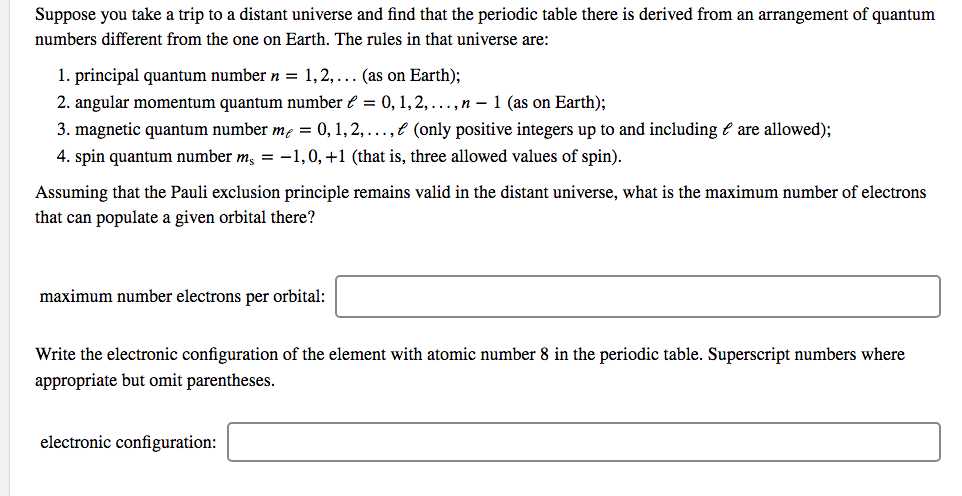The last question is what is the atomic number of the second noble gas? Suppose you take a trip to a distant universe and find that the periodic table there is derived from an arrangement of quantum numbers different from the one on Earth. The rules in that universe are: 1. principal quantum number n = 1,2,... (as on Earth); 2. angular momentum quantum number / = 0,1,2,...,n - 1 (as on Earth); 3. magnetic quantum number me = 0,1,2,...,f...

Need Online Homework Help?